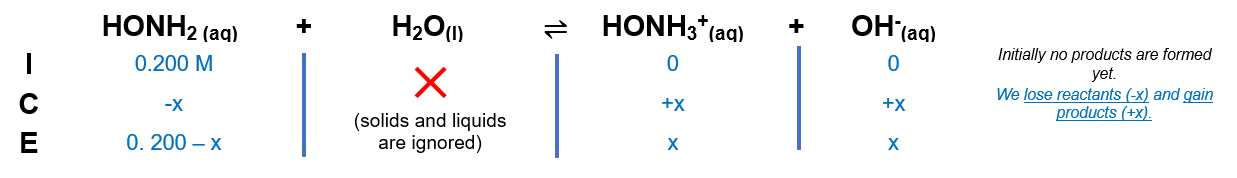# Problem: Consider the titration of 100.0 mL of 0.200 M HONH 2 by 0.100 M HCl. (K b for HONH2 = 1.1 x 10-8.)a. Calculate the pH after 0.0 mL of HCl has been added.

###### FREE Expert Solution

We are asked to calculate the pH after 0.0 mL of HCl has been added.

Since no acid is added yet, we treat is as a simple weak base.

Step 1. Set up ICE chart:Step 2. Calculate OH-

SInce Kb is small, assume x is negligible in the denominator.

x = [OH-] = 4.69 x 10-5 M

89% (477 ratings)###### Problem Details

Consider the titration of 100.0 mL of 0.200 M HONH 2 by 0.100 M HCl. (K b for HONH2 = 1.1 x 10-8.)

a. Calculate the pH after 0.0 mL of HCl has been added.